# Linear Systems of Equations Systems of Linear Equations

• Slides: 21Linear Systems of Equations • Systems of Linear Equations • Solving Systems of Equations by Graphing • Solving Systems of Equations by Substitution 1Algebra 1 Solving Systems by Graphing Objective: solve a linear system by graphing when not in slope-intercept form.Warm-Up Solve the system by graphing.Systems of Equations A set of equations is called a system of equations. The solutions must satisfy each equation in the system. If all equations in a system are linear, the system is a system of linear equations, or a linear system.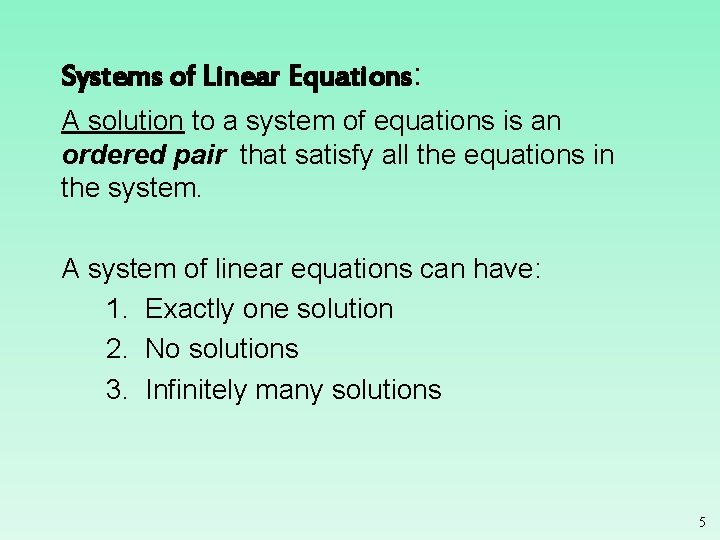Systems of Linear Equations: A solution to a system of equations is an ordered pair that satisfy all the equations in the system. A system of linear equations can have: 1. Exactly one solution 2. No solutions 3. Infinitely many solutions 5Systems of Linear Equations: There are four ways to solve systems of linear equations: 1. By graphing 2. By substitution 3. By addition (also called elimination) 4. By multiplication 6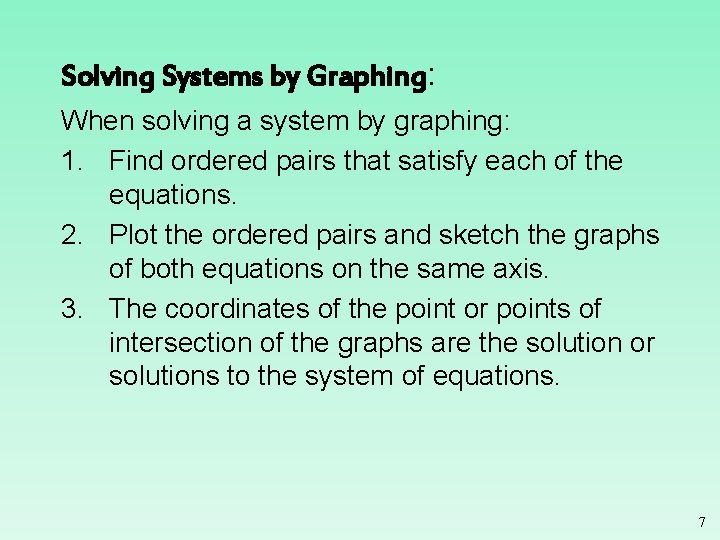Solving Systems by Graphing: When solving a system by graphing: 1. Find ordered pairs that satisfy each of the equations. 2. Plot the ordered pairs and sketch the graphs of both equations on the same axis. 3. The coordinates of the point or points of intersection of the graphs are the solution or solutions to the system of equations. 7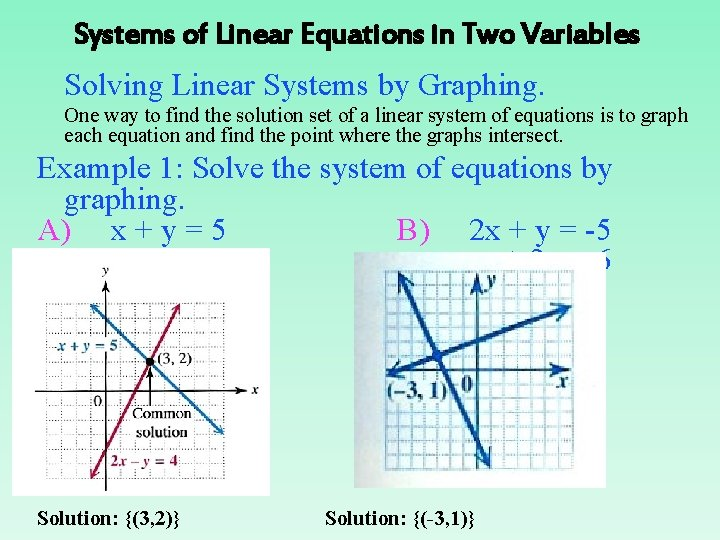Systems of Linear Equations in Two Variables Solving Linear Systems by Graphing. One way to find the solution set of a linear system of equations is to graph each equation and find the point where the graphs intersect. Example 1: Solve the system of equations by graphing. A) x + y = 5 B) 2 x + y = -5 2 x - y = 4 -x + 3 y = 6 Solution: {(3, 2)} Solution: {(-3, 1)}Deciding whether an ordered pair is a solution of a linear system. The solution set of a linear system of equations contains all ordered pairs that satisfy all the equations at the same time. Example 1: Is the ordered pair a solution of the given system? 2 x + y = -6 Substitute the ordered pair into each equation. x + 3 y = 2 Both equations must be satisfied. A) (-4, 2) B) (3, -12) 2(-4) + 2 = -6 2(3) + (-12) = -6 (-4) + 3(2) = 2 (3) + 3(-12) = 2 -6 = -6 2=2 -33 -6 Yes No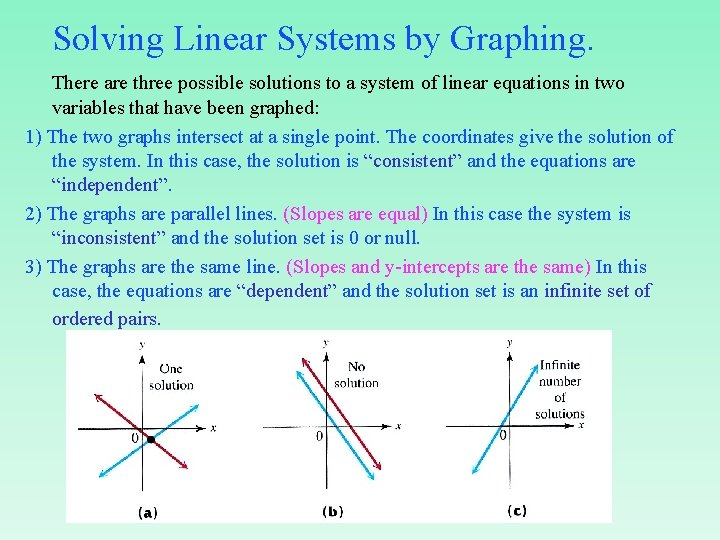Solving Linear Systems by Graphing. There are three possible solutions to a system of linear equations in two variables that have been graphed: 1) The two graphs intersect at a single point. The coordinates give the solution of the system. In this case, the solution is “consistent” and the equations are “independent”. 2) The graphs are parallel lines. (Slopes are equal) In this case the system is “inconsistent” and the solution set is 0 or null. 3) The graphs are the same line. (Slopes and y-intercepts are the same) In this case, the equations are “dependent” and the solution set is an infinite set of ordered pairs.Types of Systems • There are three possible outcomes when graphing two linear equations in a plane. • One point of intersection, so one solution • Parallel lines, so no solution • Coincident lines, so infinite # of solutions • If there is at least one solution, the system is considered to be consistent. • If the system defines distinct lines, the equations are independent.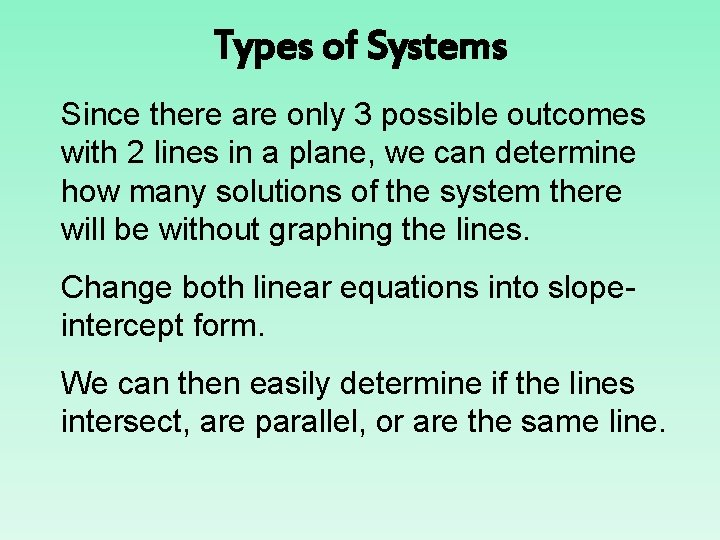Types of Systems Since there are only 3 possible outcomes with 2 lines in a plane, we can determine how many solutions of the system there will be without graphing the lines. Change both linear equations into slopeintercept form. We can then easily determine if the lines intersect, are parallel, or are the same line.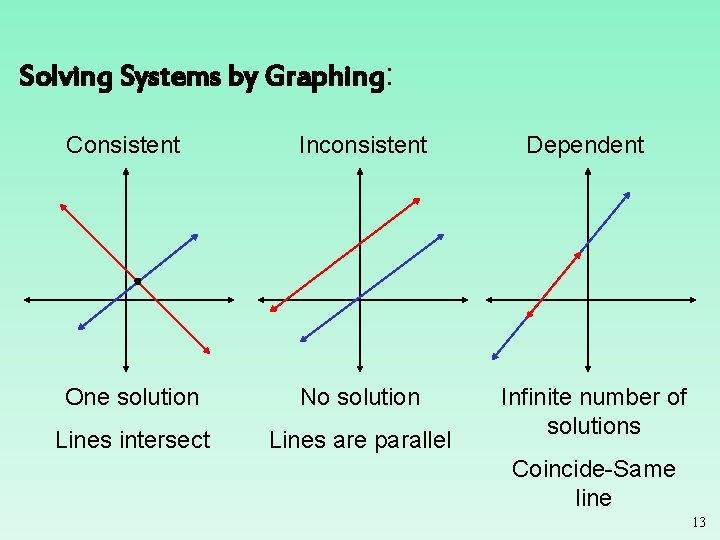Solving Systems by Graphing: Consistent Inconsistent One solution No solution Lines intersect Lines are parallel Dependent Infinite number of solutions Coincide-Same line 13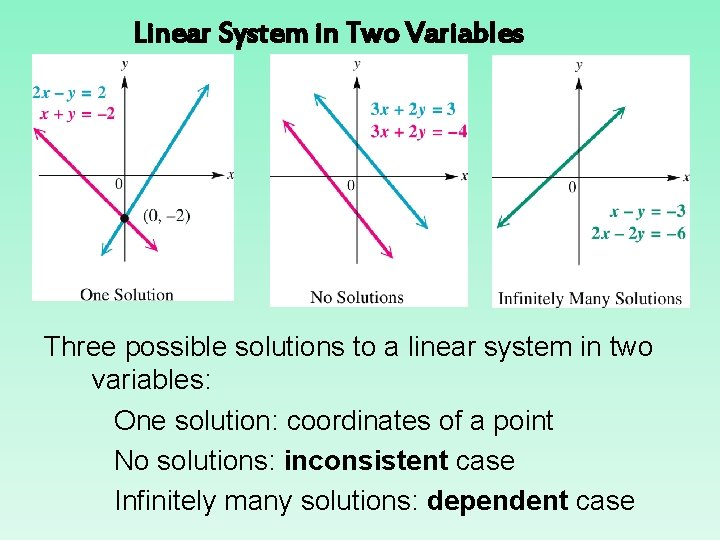Linear System in Two Variables Three possible solutions to a linear system in two variables: One solution: coordinates of a point No solutions: inconsistent case Infinitely many solutions: dependent case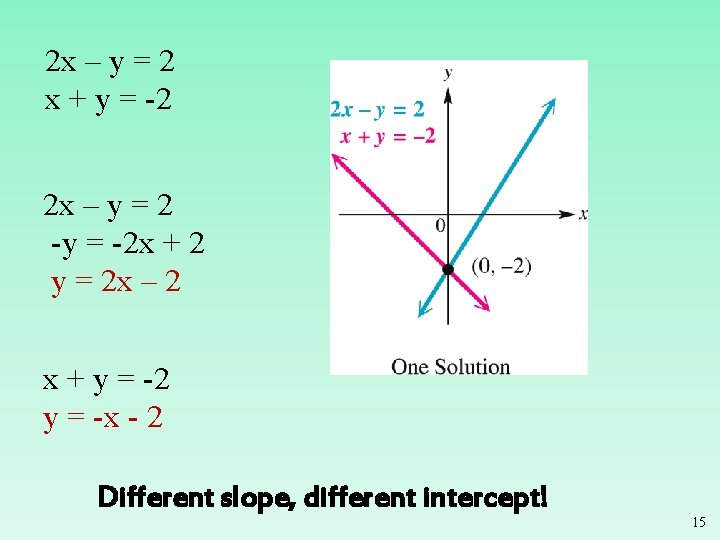2 x – y = 2 x + y = -2 2 x – y = 2 -y = -2 x + 2 y = 2 x – 2 x + y = -2 y = -x - 2 Different slope, different intercept! 153 x + 2 y = 3 3 x + 2 y = -4 3 x + 2 y = 3 2 y = -3 x + 3 y = -3/2 x + 3/2 3 x + 2 y = -4 2 y = -3 x -4 y = -3/2 x - 2 Same slope, different intercept!! 16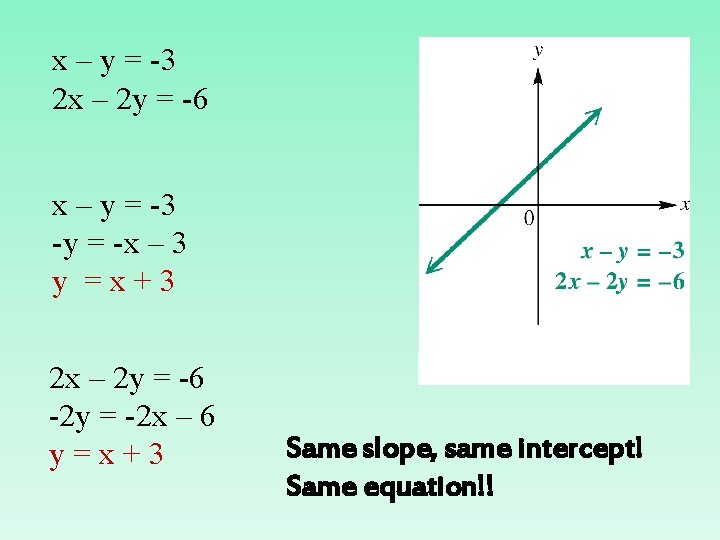x – y = -3 2 x – 2 y = -6 x – y = -3 -y = -x – 3 y =x+3 2 x – 2 y = -6 -2 y = -2 x – 6 y=x+3 Same slope, same intercept! Same equation!!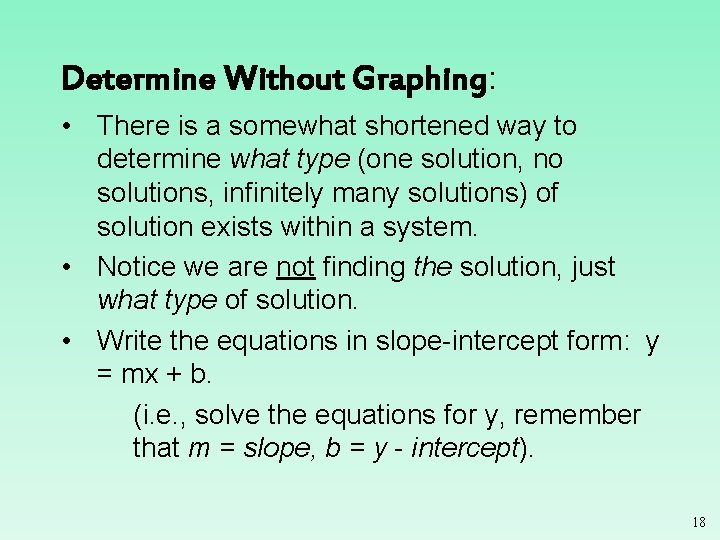Determine Without Graphing: • There is a somewhat shortened way to determine what type (one solution, no solutions, infinitely many solutions) of solution exists within a system. • Notice we are not finding the solution, just what type of solution. • Write the equations in slope-intercept form: y = mx + b. (i. e. , solve the equations for y, remember that m = slope, b = y - intercept). 18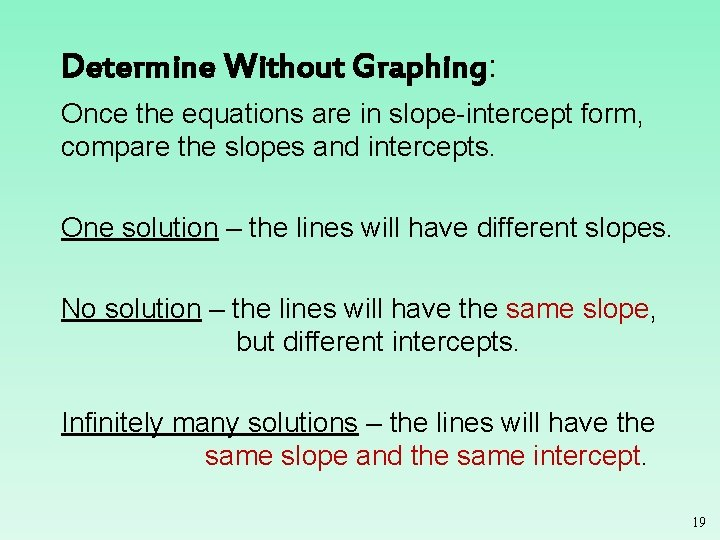Determine Without Graphing: Once the equations are in slope-intercept form, compare the slopes and intercepts. One solution – the lines will have different slopes. No solution – the lines will have the same slope, but different intercepts. Infinitely many solutions – the lines will have the same slope and the same intercept. 19Determine Without Graphing: Given the following lines, determine what type of solution exists, without graphing. Equation 1: 3 x = 6 y + 5 Equation 2: y = (1/2)x – 3 Writing each in slope-intercept form (solve for y) Equation 1: y = (1/2)x – 5/6 Equation 2: y = (1/2)x – 3 Since the lines have the same slope but different y-intercepts, there is no solution to the system of equations. The lines are parallel. 20Class Work Solve the system by graphing.